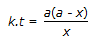# Chemical Engineering - Chemical Reaction Engineering

### Exercise :: Chemical Reaction Engineering - Section 10

26.

Pick out the correct statement.

 A. A catalyst speeds up the forward reaction but slows shown the reverse reaction. B. Addition of catalyst changes the equilibrium constant. C. Pressure changes do not change the equilibrium concentrations. D. The composition of equilibrium is changed by catalyst.

Explanation:

No answer description available for this question. Let us discuss.

27.

In which of the following gaseous phase reactions, the equilibrium of the reaction remains unaffacted by pressure changes ?

 A. 2O3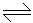3O2 B. N2 + O22NO C. 2NO2N2O4 D. 2SO2 + O22SO3

Explanation:

No answer description available for this question. Let us discuss.

28.

The mean conversion in the exit stream, for a second order, liquid phase reaction in a non-ideal flow reactor is given by

 A.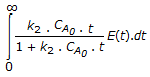B.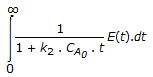C.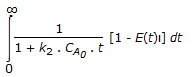D.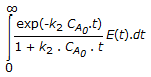Explanation:

No answer description available for this question. Let us discuss.

29.

Integral method for analysing the kinetic data is used

 A. when the data are scattered. B. for testing specific mechanisms with simple rate expressions. C. both (a) and (b). D. none of these.

Explanation:

No answer description available for this question. Let us discuss.

30.

Concentration of the limiting reactant (with initial concentration of a moles/litre) after time t is (a-x). Then 't' for a first order reaction is given by

 A.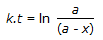B.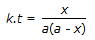C.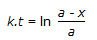D.